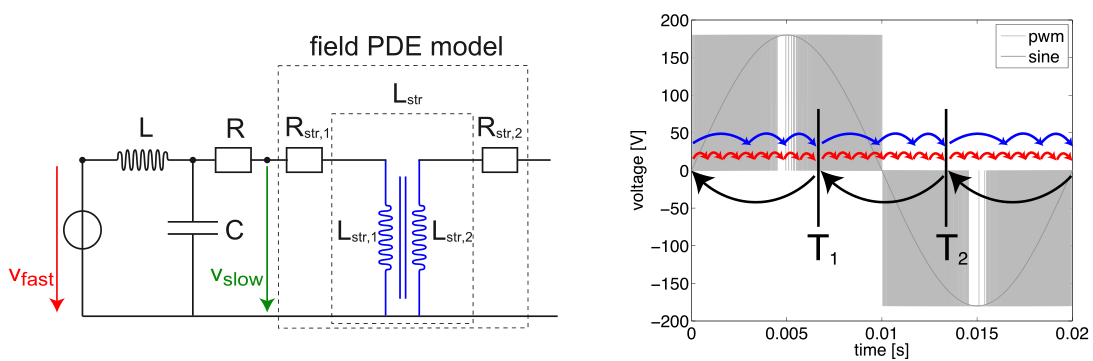# Co-simulation & UQ within the SIMUROM project

In many applications, coupled problems appear very naturally. For example, Fig.1 depicts a field/circuit problem, where a transformer can be modeled by a partial differential equation (PDE) and an electric circuit subsystem, which can be modeled by differential algebraic equations (DAEs). The coupled system is a PDAE . A semi-discretization in space yields a system of coupled DAEs. We remark that the inherent time scales of the subsystems may differ largely.

Now, co-simulation is an important method for the transient analysis of such coupled systems. In electrical engineering, for example, it traces back to the seminal approach discussed in . In particular, if a monolithic description of a dynamical system is not feasible and/or dedicated simulation tools for the corresponding subsystems are available, it can be very useful.Fig.1: Field/circuit coupling and pulse width modulated signal, .

How does it work? Usually, the simulation time is split into time windows on which the subsystems are solved sequentially. Starting from an extrapolation, the waveforms of the coupling variables are frozen and thus the subsystems can be solved separately. If needed, the procedure can be iterated, where the latest computed solution describes the frozen coupling waveforms. Thus co-simulation  combines multirate, multimethod and multiorder features automatically. Compare with Fig.1 (right). In mathematics the method is also called dynamic iteration or waveform iteration, which underlines that it is a fixed-point iteration.

When does it work? In contrast to coupled ordinary differential equations (ODEs), co-simulation of differential-algebraic equations (DAEs) is not guaranteed to converge. In the DAE case, a contraction condition is needed, see e.g. . It can be shown that the convergence and stability of co-simulation is directly influenced by the order of computation and by the coupling interface, see e.g. .

The SIMUROM project is funded by the German Ministry on Education and Research (BMBF) within the program on “Mathematics for innovations in industry and services” (see http://www.simurom.de/). Within this project, we analyze the design of coupling interfaces especially for field/circuit coupled systems in order to improve the convergence speed within the co-simulation algorithm . Often model data suffers from uncertainties in parameters, design etc. To this end, we investigate the impact of submodel uncertainty to the co-simulation procedure, where uncertain parameters are described as random variables with given distribution functions. Thus the contraction factor becomes a random variable, and the convergence of the co-simulation approach is linked to the distribution function of the contraction factor.

Kai Gausling & Andreas Bartel

References
 Arnold, M., Günther, M.: Preconditioned Dynamic Iteration for Coupled Differential-Algebraic Systems. BIT, vol. 41, pp. 1–25 (2001).
 Bartel, A., Brunk, M., Schöps, S.: On the convergence rate of dynamic iteration for coupled problems with multiple subsystems. J. Comput. Appl. Math., vol. 262, pp. 14–24 (2014).
 Gausling, K., Bartel, A.: Coupling interfaces and their impact in Field/Circuit Co-Simulation. Accepted at IEEE Transactions on Magnetics (2015).
 Lelarasmee, E., Ruehli, A. E., Sangiovanni-Vincentelli, A.: The Waveform Relaxation Method for Time Domain Analysis of Large Scale Integrated Circuits. IEEE Computer-Aided Design of Integrated Circuits and Systems, vol. 1, pp. 131 – 145 (1982).
 Schöps, S.: Multiscale Modeling and Multirate Time-integration of Field/circuit Coupled Problems. Ph.D. thesis, Bergische Universität Wuppertal (2011).